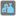• # Weak checker since it uses a common scale

## Question related to missionBag of Santa Claus

Right now, the checker define a scale, then do 2000 tests.

Problem is after a few tests and thanks to global variables (which are necessary for the task), we can have a really good estimation of the scale. Then if we know the scale, the problem become much easier. And wrong solutions can pass tests.

To fix the issue here, two solutions: clear user global variables between each test (I'm not sure how... so forgot it...), or way easier define a scale for each of the 2000 tests (it only requires to move a line in the checker).

```scale = (random() + random()) ** randint(0, 1024)
...
for i in ...:
...
```

Become

```for i in ...:
scale = (random() + random()) ** randint(0, 1024)
...
```

Then there is no common scale to the 2000 tests. We still can have an estimation of it (if necessary) but not know a TOO good one.

I'm just a bit surprised it was not detected before.

PS: By the way, I think it is a weird way to define a scale.48